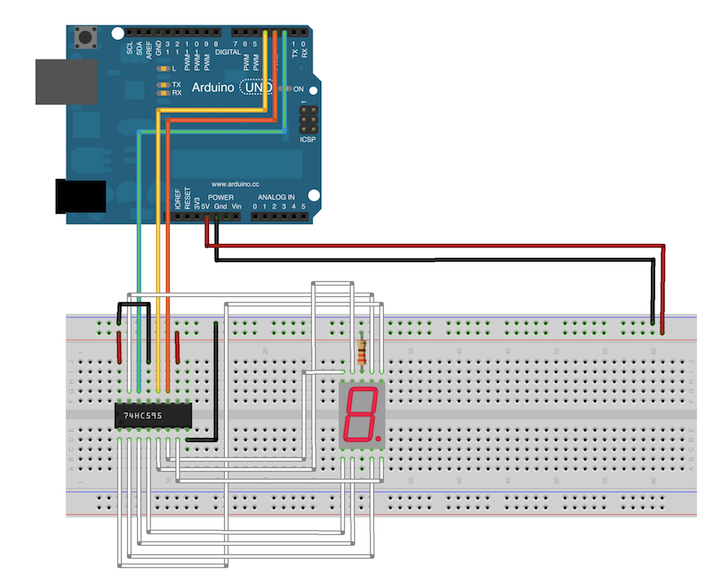##### Breadboard for "Shift Register - Seven Segment controller"Fritzing diagram: shift-register-seven-segment.fzz

Run this example from the command line with:

``````node eg/shift-register-seven-segment.js
``````
``````const {Board, ShiftRegister} = require("johnny-five");
const board = new Board();

const register = new ShiftRegister({
pins: {
data: 2,
clock: 3,
latch: 4,
}
});
let number = 0;
let decimal = 0;

// Display numbers 0-9, one at a time in a loop.
// Shows just the number for a half second, then
// the number + a decimal point for a half second.
setInterval(() => {
register.display(number + (decimal && "."));

if (decimal) {
number++;
}

if (number === 10) {
number = 0;
}

decimal ^= 1;
}, 500);
});

``````

### Component Classes in this example:

Hi! The Johnny-Five community is building new projects every day. We made this newsletter to tell you about what's new, what's good, and what's next for Open Source robotics. Join us in exploring what we can make together.